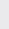Course Content

0/4

0/3

0/1

0/2

0/1

0/4

0/3

0/5

0/3

0/5

0/5

0/1

0/6

0/2

0/4

0/5

11.1 Cartesian System Coordinate 直角坐标系

(1) Find the possible values of m if the distance between P(3, m) and Q(0, 2) is 5.

m 所有值，若 P(3, m) 和 Q(0, 2) 之间的距离是 5.

6, -2

(2) Given that P(h, 3), Q(-2, 1) and R(3, 2). Calculate all the possible values of h if distance of PQ is twice of the distance of QR.

-12, 8

(3) A(a, 3), B(1, -1), C(-2, b) and D(0, 2) are the vertices of a parallelogram. Find the values of a and b.

A(a, 3), B(1, -1), C(-2, b) 和 D(0, 2) 是一个平行四边形的顶点. 求 a 和 b之值.

3, -2

(4) A(2, -3), B(h, k) and C(-3, 4) three points are located at the same straight line such that 5AB = 2BC. Find the values of h and k.

A(2, -3), B(h, k) 和 C(-3, 4) 三点在一条线上的点且5AB = 2BC. 求 h 和 k的., -1

(5) Points A(4, 9), B(-1, -3) and C(-8, 4), show that AB = AC.
If P(a, b) is a point which has the same distance from B and C, prove that a = b – 5.

(6) The distance from (-a, a) to the midpoint of P(-3, -3) and Q(-7, -7) is 10 units, find the value of a.